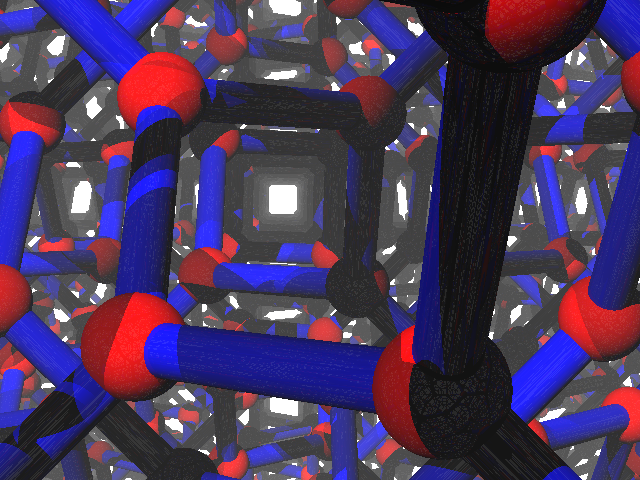The crystallographers must have a name for this pattern but I don't know what it is — maybe someone can fill me in? Start with the 16 points

    (0,0,0), (2,1,0), (1,2,0), (3,3,0),
(0,1,1), (2,0,1), (1,3,1), (3,2,1),
(1,1,2), (3,0,2), (0,3,2), (2,2,2),
(1,0,3), (3,1,3), (0,2,3), (2,3,3)

and then repeat in a cubical grid pattern by adding arbitrary multiples of four to each coordinate. Connect every two points at distance $$\sqrt{2}$$ in the resulting infinite point set.

In the resulting pattern, every point has exactly three neighbors and the angles between its three incident edges are exactly 120 degrees. There are also many square helices parallel to each of the three coordinate directions, e.g. (0,0,0), (0,1,1), (1,1,2), (1,0,3), (0,0,4), (0,1,5), ... or (2,1,0), (1,2,0), (1,3,1), (2,4,1), (2,5,0), (1,6,0), ... Here's an image looking directly down one of these helices.When viewed as an infinite graph, this actually has two connected components, interleaved with each other. Each has chiral symmetry (the square helices in one of the components wind clockwise, the other counterclockwise) and they fit together to form a more symmetric structure with twice the density of each component.

Unfortunately I don't think carbon crystals will take a form like this because the edge planes of two adjacent vertices in this structure are twisted with respect to each other rather than coplanar which seems not to be energetically favorable. But maybe some other crystal unit allows that kind of twisting and still likes having 120 degree angles?